# Solution assignment 01 Quadratic equations (abc-formula)

### Assignment 1

Solve:### Solution

This equation can be solved quite easily by factorizing.
We write: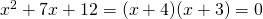and thus the solutions are: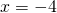or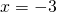If you want to use the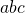-formula we take the famous-formula: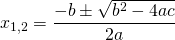In this case: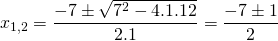and thus the solutions are: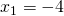or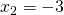which are equal to the solutions we found earlier.

0